# RD Sharma Class 10 Solutions Chapter 11 Trigonometric Identities VSAQS

## RD Sharma Class 10 Solutions Chapter 11 Trigonometric Identities VSAQS

These Solutions are part of RD Sharma Class 10 Solutions. Here we have given RD Sharma Class 10 Solutions Chapter 11 Trigonometric Identities VSAQS

Other Exercises

Answer each of the following questions either in one word or one sentence or as per requirement of the questions :
Question 1.
Define an identity.
Solution:
An identity is an equation which is true for all values of the variable (s) involved.

Question 2.
What is the value of (1 – cos2 θ) cosec2 θ?
Solution: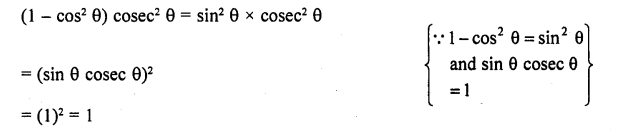Question 3.
What is the value of (1 + cot2 θ) sin2 θ ?
Solution:
(1 + cot2 θ) sin2 θ = cosec2 sin2 θ                           {1 + cot2 θ = cosec2 θ}
= (cosec θ sin θ)2 = (l)2 = 1                                      (∵ sin θ cosec θ=1)

Question 4.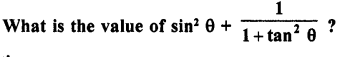Solution: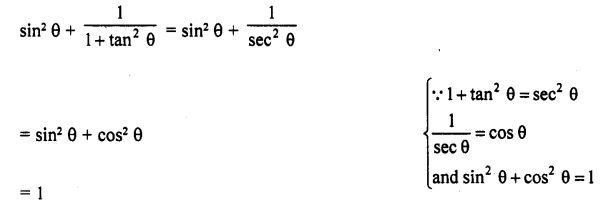Question 5.
If sec θ + tan θ = x, write the value of sec θ – tan θ in terms of x.
Solution:
sec θ+ tan θ = x
We know that
sec2 θ – tan2 θ=1                                                                    .
⇒ (sec θ + tan θ) (sec θ – tan θ) = 1                               {a2 – b2 = (a + b) (a – b)}
⇒  x (sec θ – tan θ) = 1
⇒  sec θ – tan θ = $$\frac { 1 }{ x }$$

Question 6.
If cosec θ – cot θ = α, write the value of cosec θ + cot α.
Solution:
cosec θ – cot θ = α
We know that,
cosec θ – cot θ=1
⇒  (cosec θ – cot θ) (cosec θ + cot θ) = 1
⇒  a (cosec θ + cot θ) = 1                                                {a2 – b2 = (a + b) (a – 6)}
⇒  cosec θ + cot θ = 1/α

Question 7.
Write the value of cosec2 (90° – θ) – tan2θ
Solution: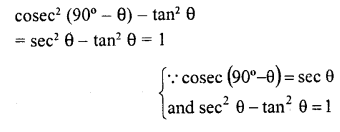Question 8.
Write the value of sin A cos (90° – A) + cos A sin (90° – A)
Solution: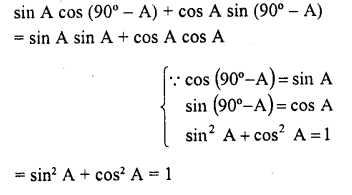Question 9.Solution: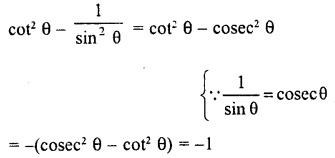Question 10.
If x = a sin θ and y = b cos θ, what is the value of b2x2 + a2y2 ?
Solution:
x = a sin θ, y = b cos θ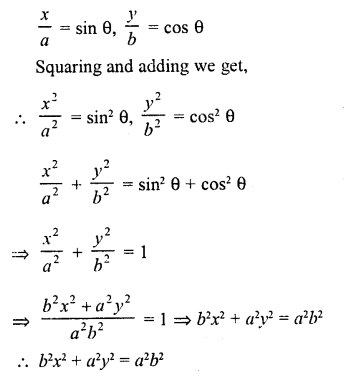Question 11.
If sin θ = $$\frac { 4 }{ 5 }$$, What is the value of cot θ + cosec θ ?
Solution: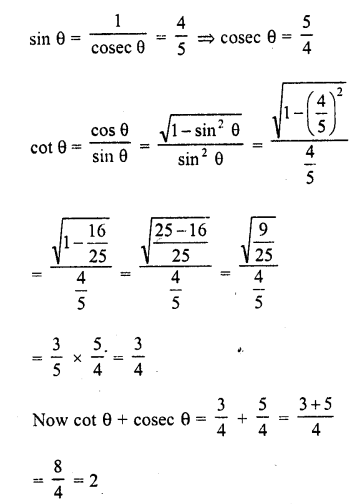Question 12.
What is the value of 9 cot θ-9 cosec θ?
Solution:
9 cot θ – 9 cosec θ
= -(9cosec θ – 9cot θ)
= -9 (cosec θ – cot2 θ) = -9 x 1
= -9                                                       {∵  cosec θ – cot2 θ=1}

Question 13.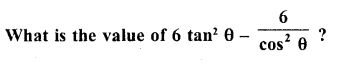Solution: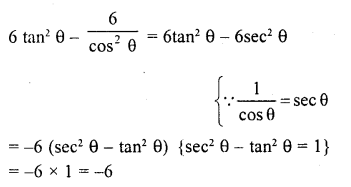Question 14.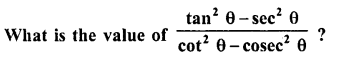Solution: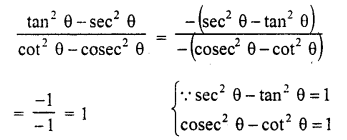Question 15.
What is the value of (1 + tan2 θ) (1 – sin θ) (1 + sin θ) ?
Solution: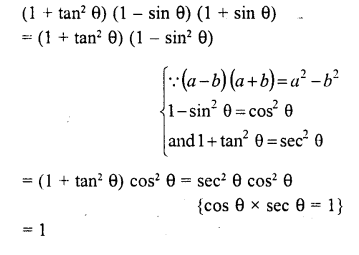Question 16.
If cos A = $$\frac { 7 }{ 25 }$$, find the value of tan A +cot A. (C.B.S.E. 2008)
Solution: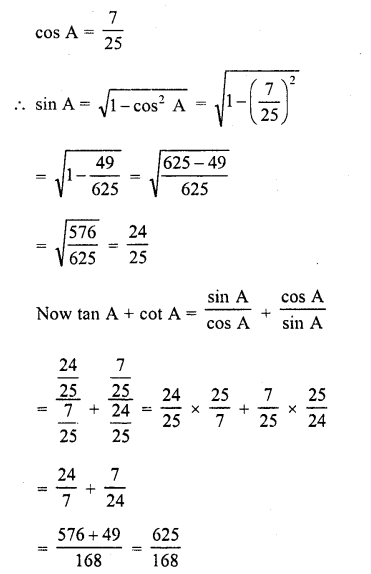Question 17.
If sin θ = $$\frac { 1 }{ 3 }$$, then find the value of 2 cot2 θ + 2(C.B.S.E. 2009)
Solution: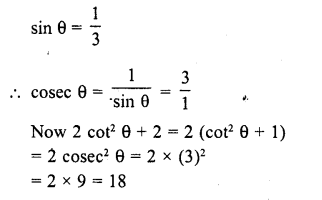Question 18.
If cos θ = $$\frac { 3 }{ 4 }$$, then find the value of 9 tan2 θ + 9.
Solution: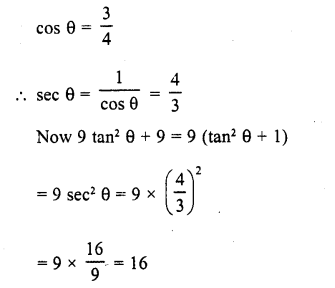Question 19.
If sec θ (1 + sin θ) (1 – sin θ) = k, then find the value of k. (C.B.S.E. 2009)
Solution: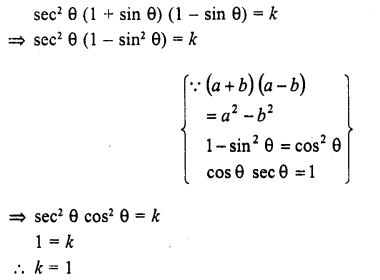Question 20.
If cosec θ (1 + cos θ) (1 – cos θ) = λ, then find the value of λ.
Solution:
cosec θ (1 + cos θ) (1 – cos θ) = λ
⇒ cosec2 θ (1 – cos2 θ) = λ                      {(a + b) (a – b) = a1 – b2)}
⇒  cosec2 θ x sin θ = λ             (1 – cos2 θ = sin2 θ)
⇒ 1 = λ                                      (sin θ cosec θ=1)
∴ λ = 1

Question 21.
If sin2 θ cos2 θ (1 + tan2 θ) (1 + cot2 θ) = λ, then find the value of λ.
Solution:
sin2 θ cos2 θ (1+ tan2 θ) (1 + cot2 θ) = λ
sin2 θ cos2 θ (sec2 θ) (cosec2 θ) = λ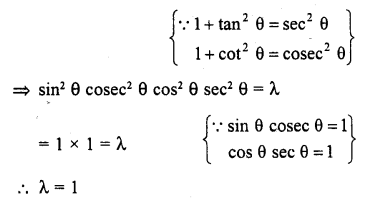Question 22.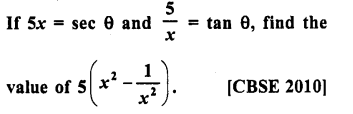Solution: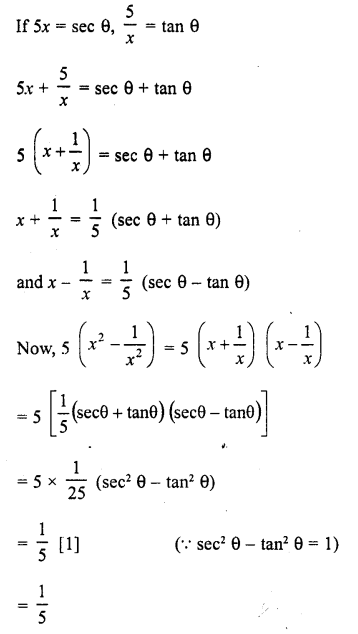Question 23.
If cosec θ = 2x and cot θ = $$\frac { 2 }{ x }$$, find the value of 2 ( x2 – $$\frac { 1 }{ x2 }$$           [CBSE 2010]
Solution: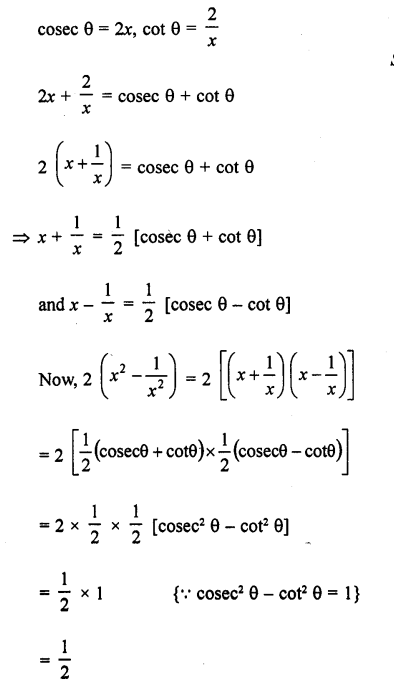Question 24.
Write ‘True’ or ‘False’ and justify your answer in each of the following:
(i) The value of sin θ is x + $$\frac { 1 }{ x }$$, where ‘x’ is a positive real number.

(ii) cos θ = $$\frac { { a }^{ 2 }+{ b }^{ 2 } }{ 2ab }$$ , where a and b are two lab distinct numbers such that ab > 0.
(iii) The value of cos2 23 – sin2 67 is positive.
(iv) The value of the expression sin 80° – cos 80° is negative.
(v) The value of sin θ + cos θ is always greater than 1.
Solution: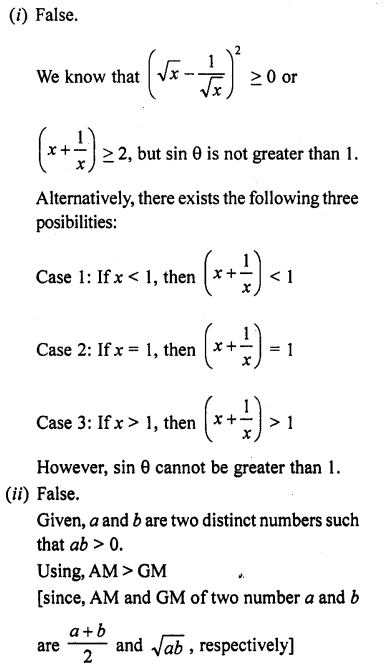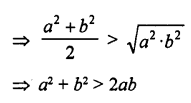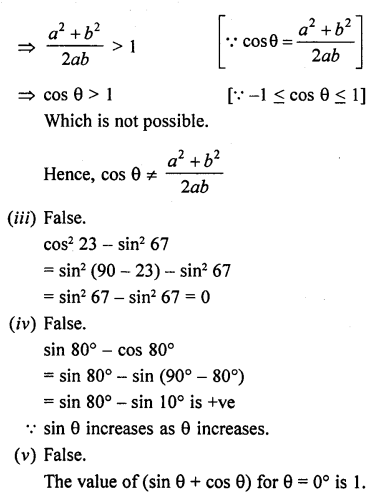Hope given RD Sharma Class 10 Solutions Chapter 11 Trigonometric Identities VSAQS are helpful to complete your math homework.

If you have any doubts, please comment below. Learn Insta try to provide online math tutoring for you.